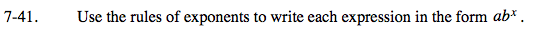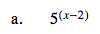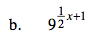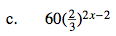### Home > PC > Chapter 7 > Lesson 7.2.1 > Problem7-41

7-41.5x · 5 −2$\left (3^{2} \right )^{\frac{1}{2}x+1}$$60\cdot \left (\frac{2}{3}\right)^{2x}\cdot \left(\frac{2}{3}\right)^{-2}$